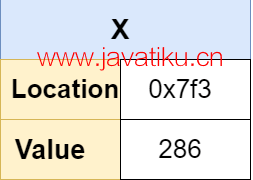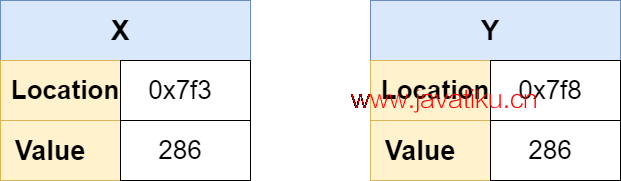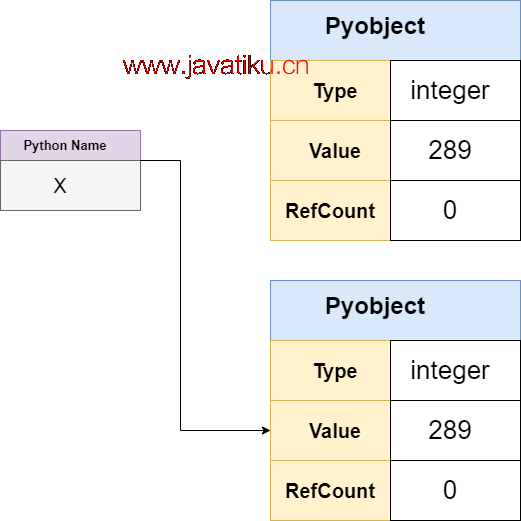# Tkinter 教程-Python中的指针 | 为什么Python不支持指针## 什么是指针？

"指针是存储另一个变量的内存地址的变量。指针变量用星号（*）表示。"

``````#include <stdio.h>
int main()
{
int* po, o;

0 = 10;
printf("Value of c: %d\n\n", c);

o = &0;
printf("Address of pointer pc: %p\n", o);
printf("Content of pointer pc: %d\n\n", *o);
0 = 11;
printf("Address of pointer pc: %p\n", p0);
printf("Content of pointer pc: %d\n\n", *p0);
*po = 2;
printf("Value of c: %d\n\n", o);
return 0;
}  ``````

``````Address of o: 2686784
Value of o: 22

Content of pointer po: 22

Content of pointer po: 11

Value of o: 2``````

## 为什么Python不支持指针

• 不可变对象 vs. 可变对象
• Python变量/名称

• 引用计数
• 类型

## 不可变对象 vs. 可变对象

``````x = 5
id(x)  ``````

``140720979625920``

``````x-=1
id(x)``````

``140720979625888``

``````s = "java"
print(id(s))

s += "Tiku"
print(s)

id(s)``````

``````2315970974512
Javatiku
1977728175088``````

``````s = 'java'
s = T
print(id(s))``````

``````Traceback (most recent call last):
File "C:/Users/DEVANSH SHARMA/PycharmProjects/MyPythonProject/python1.py", line 34, in
s = T
NameError: name 'T' is not defined``````

``````my_list = [3, 4, 8]
print(id(my_list))

my_list.append(4)
print(my_list)

print(id(my_list))``````

``````2571132658944
[3, 4, 8, 4]
2571132658944``````

## 理解Python变量

### C中的变量

``int x = 286;``
• 为整数分配足够的内存。
• 将值286分配给该内存位置。
• x表示该值。x = 250

``````cCopy code
int y = x;``````### Python中的名称

``x = 289``

1. 创建一个PyObject。
2. 为PyObject设置整数的类型代码。
3. 为PyObject设置值289。
4. 创建一个名为x的名称。
5. 将x指向新的PyObject。
6. 将PyObject的引用计数增加1。``y = x``

``y is x ``

``True``

``````y += 1
y is x``````

## 在Python中模拟指针

• 使用可变类型作为指针
• 使用自定义Python对象

### 使用可变类型作为指针

C

``````void add_one(int *a) {
*a += 1;
} ``````

``````#include <stdio.h>

int main(void) {
int y = 233;
printf("y = %d\n", y);
printf("y = %d\n", y);
return 0;
}  ``````

``````y = 233
y = 234``````

``````def add_one(x):
x += 1

y = 
y  ``````

``````z = (2337,)

``````Traceback (most recent call last):
File "", line 1, in
File "", line 2, in add_one
TypeError: 'tuple' object does not support item assignment``````

``````count = {"funcCalls": 0}
def car():
count["funcCalls"] += 1

def foo():
count["funCcalls"] += 1
car()

foo()
count["funcCalls"] ``````

``2``

### 使用Python对象

``````class Pointer(object):
def __init__(self):
self._metrics = {
"funCalls": 0,
"catPictures": 0,
}  ``````

``````class Pointer(object):
# ...

@property
def funCalls(self):
return self._metrics["func_calls"]

@property
def catPictures_served(self):
return self._metrics["cat_pictures_served"]``````

``````pt = Pointer()
pt.funCalls()
pt.catPicture_served  ``````

``````class Pointer(object):
# ...

def increament(self):
self._metrices["funCalls"] += 1

def cat_pics(self):
self._metrices["catPictures_served"] += 1``````

``````pt = Pointer()
pt.increment()
pt.increment()
pt.funCalls()``````

## Python ctypes 模块

Python ctypes 模块允许我们在Python中创建C类型的指针。如果我们想要调用一个需要指针的C库函数，这个模块非常有用。让我们来理解以下示例。

``````void incr_one(int *x) {
*x += 1;
}``````

``````\$ gcc -c -Wall -Werror -fpic incrPointer.c
\$ gcc -shared -o libinc.so incrPointer.o  ``````

``````import ctypes
##  libinc.so library should be same directory as this program
lib = ctypes.CDLL("./libinc.so")
lib.increment  ``````

``<_FuncPtr object at 0x7f46bf6e0750>``

``````inc = lib.increment
## defining the argtypes
inc.argtypes = [ctypes.POINTER(ctypes.c_int)]``````

``incrPointer(10)  ``

``````Traceback (most recent call last):
File "<stdin>", line 1, in <module>
ctypes.ArgumentError: argument 1: <class 'TypeError'>: expected LP_c_int instance instead of int``````

``v = ctypes.c_int(10)``

v是一个C变量。ctypes提供了名为byref()的方法，用于传递变量的引用。

``````inc(ctypes.byref(a))
a``````

``c_int(11)``